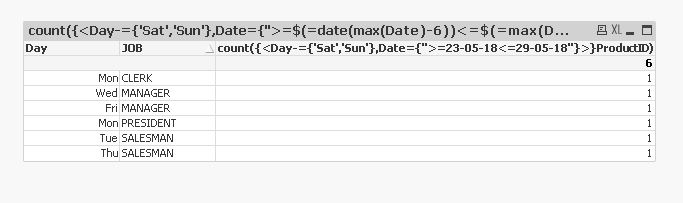# New to QlikView

Discussion board where members can get started with QlikView.

Announcements
The #1 reason QlikView customers adopt Qlik Sense is a desire for a modern BI experience. Read More
cancel
Showing results for
Did you mean:
HighlightedCreator II

## Show data from last 7 days except Saturday and Sunday (Set Analysis)

Hi Community,

I'm trying to create one expression where the result can show the current + last 7 days except for Saturday and Sunday.

My current formula is:

Today:

Count({<%Date = {"\$(=date(OrderDate,'YYYY-MM-DD'))"}>} Distinct ProductID)

Yesterday:

Count({<%Date = {"\$(=date(OrderDate-1,'YYYY-MM-DD'))"}>} Distinct ProductID)

Ideal solution:

One formula.

1 Solution

Accepted Solutions
HighlightedMaster II

Hi,

Try like this.

count({<Day-={'Sat','Sun'},Date={">=\$(=date(max(Date)-6))<=\$(=max(Date))"}>}ProductID)4 Replies
HighlightedPartner

Hi M,

There are a few different ways you can do this.

I have attached a sample which uses the weekstart and weekend functions to set date variables and then in set analysis I have picked up the date range but excluded Saturdays and Sundays as per my calendar.

The end result is a single formula which is expressed as:

=count({\$<WeekDay-={Sat, Sun}, Date={">=\$(vStartDate)<=\$(vEndDate)"}>}distinct ProductId)

Hopefully this helps.

Rod

HighlightedMVP

I guess lastworkdate() is what you need. Try something like:

Count({<%Date = {" >=\$(=today()) <=\$(=date(lastworkdate(today(),7),'YYYY-MM-DD'))"}>} Distinct ProductID)

HighlightedMaster II

Hi,

Try like this.

count({<Day-={'Sat','Sun'},Date={">=\$(=date(max(Date)-6))<=\$(=max(Date))"}>}ProductID)HighlightedCreator

Create a weekday(OrderDate)  as OrderDateWeekDays field in the backend  and use the expression

=count({<OrderDate={">=\$(=max(OrderDate)-6) <=\$(=max(OrderDate))"},OrderDateWeekDays={'Mon','Tue','Wed','Thu','Fri','Sat'}>} Distinct ProductID)